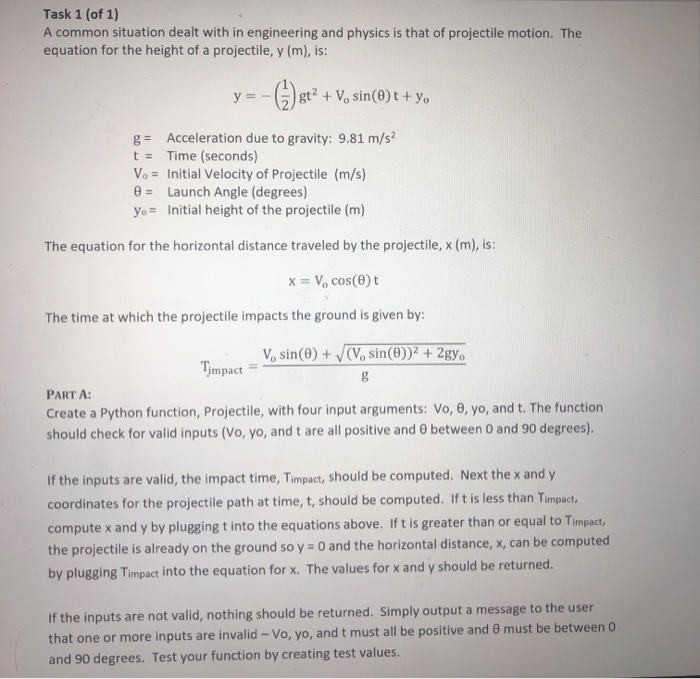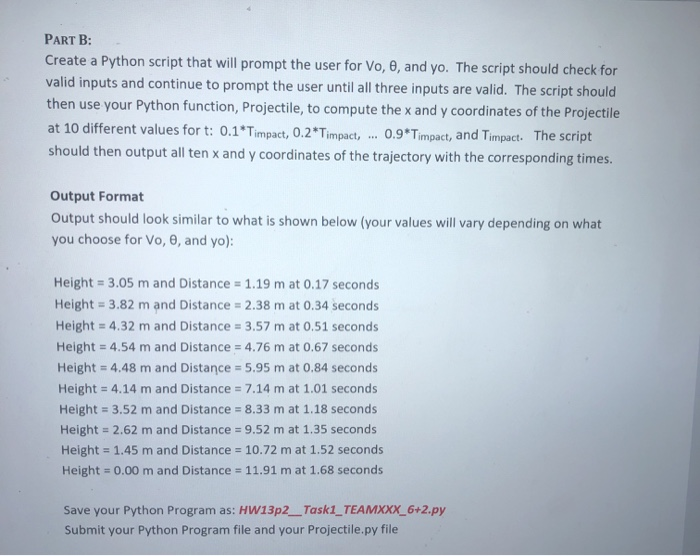# (Solved) : Task 1 1 Common Situation Dealt Engineering Physics Projectile Motion Equation Height Proj Q42777452 . . .Task 1 (of 1) A common situation dealt with in engineering and physics is that of projectile motion. The equation for the height of a projectile, y (m), is: V, sin()t + yo g= Acceleration due to gravity: 9.81 m/s2 t = Time (seconds) Vo = Initial Velocity of Projectile (m/s) 0 = Launch Angle (degrees) yo = Initial height of the projectile (m) The equation for the horizontal distance traveled by the projectile, x (m), is: X = V, cost The time at which the projectile impacts the ground is given by: V, sin(0) + (V.sin(O))2 + 2gy. Timpact = PART A: Create a Python function, Projectile, with four input arguments: Vo, e, yo, and t. The function should check for valid inputs (vo, yo, and t are all positive and between 0 and 90 degrees). If the inputs are valid, the impact time, Timpact, should be computed. Next the x and y coordinates for the projectile path at time, t, should be computed. Ift is less than Timpact, compute x and y by pluggingt into the equations above. Ift is greater than or equal to Timpact, the projectile is already on the ground so y = 0 and the horizontal distance, x, can be computed by plugging Timpact into the equation for x. The values for x and y should be returned. If the inputs are not valid, nothing should be returned. Simply output a message to the user that one or more inputs are invalid -Vo, yo, and t must all be positive and must be between 0 and 90 degrees. Test your function by creating test values. PART B: Create a Python script that will prompt the user for Vo, 0, and yo. The script should check for valid inputs and continue to prompt the user until all three inputs are valid. The script should then use your Python function, Projectile, to compute the x and y coordinates of the Projectile at 10 different values for t: 0.1* Timpact, 0.2*Timpact, … 0.9*Timpact, and Timpact. The script should then output all ten x and y coordinates of the trajectory with the corresponding times. Output Format Output should look similar to what is shown below (your values will vary depending on what you choose for Vo, e, and yo): Height = 3.05 m and Distance = 1.19 m at 0.17 seconds Height = 3.82 m and Distance = 2.38 m at 0.34 seconds Height = 4.32 m and Distance = 3.57 m at 0.51 seconds Height = 4.54 m and Distance = 4.76 m at 0.67 seconds Height = 4.48 m and Distance = 5.95 m at 0.84 seconds Height = 4.14 m and Distance = 7.14 m at 1.01 seconds Height = 3.52 m and Distance = 8.33 m at 1.18 seconds Height = 2.62 m and Distance = 9.52 m at 1.35 seconds Height = 1.45 m and Distance = 10.72 m at 1.52 seconds Height = 0.00 m and Distance = 11.91 mat 1.68 seconds Save your Python Program as: HW13p2__ Task1_TEAMXXX_6+2.py Submit your Python Program file and your Projectile.py file Show transcribed image text Task 1 (of 1) A common situation dealt with in engineering and physics is that of projectile motion. The equation for the height of a projectile, y (m), is: V, sin()t + yo g= Acceleration due to gravity: 9.81 m/s2 t = Time (seconds) Vo = Initial Velocity of Projectile (m/s) 0 = Launch Angle (degrees) yo = Initial height of the projectile (m) The equation for the horizontal distance traveled by the projectile, x (m), is: X = V, cost The time at which the projectile impacts the ground is given by: V, sin(0) + (V.sin(O))2 + 2gy. Timpact = PART A: Create a Python function, Projectile, with four input arguments: Vo, e, yo, and t. The function should check for valid inputs (vo, yo, and t are all positive and between 0 and 90 degrees). If the inputs are valid, the impact time, Timpact, should be computed. Next the x and y coordinates for the projectile path at time, t, should be computed. Ift is less than Timpact, compute x and y by pluggingt into the equations above. Ift is greater than or equal to Timpact, the projectile is already on the ground so y = 0 and the horizontal distance, x, can be computed by plugging Timpact into the equation for x. The values for x and y should be returned. If the inputs are not valid, nothing should be returned. Simply output a message to the user that one or more inputs are invalid -Vo, yo, and t must all be positive and must be between 0 and 90 degrees. Test your function by creating test values.
PART B: Create a Python script that will prompt the user for Vo, 0, and yo. The script should check for valid inputs and continue to prompt the user until all three inputs are valid. The script should then use your Python function, Projectile, to compute the x and y coordinates of the Projectile at 10 different values for t: 0.1* Timpact, 0.2*Timpact, … 0.9*Timpact, and Timpact. The script should then output all ten x and y coordinates of the trajectory with the corresponding times. Output Format Output should look similar to what is shown below (your values will vary depending on what you choose for Vo, e, and yo): Height = 3.05 m and Distance = 1.19 m at 0.17 seconds Height = 3.82 m and Distance = 2.38 m at 0.34 seconds Height = 4.32 m and Distance = 3.57 m at 0.51 seconds Height = 4.54 m and Distance = 4.76 m at 0.67 seconds Height = 4.48 m and Distance = 5.95 m at 0.84 seconds Height = 4.14 m and Distance = 7.14 m at 1.01 seconds Height = 3.52 m and Distance = 8.33 m at 1.18 seconds Height = 2.62 m and Distance = 9.52 m at 1.35 seconds Height = 1.45 m and Distance = 10.72 m at 1.52 seconds Height = 0.00 m and Distance = 11.91 mat 1.68 seconds Save your Python Program as: HW13p2__ Task1_TEAMXXX_6+2.py Submit your Python Program file and your Projectile.py file

Answer to Task 1 (of 1) A common situation dealt with in engineering and physics is that of projectile motion. The equation for th…

We are the best freelance writing portal. Looking for online writing, editing or proofreading jobs? We have plenty of writing assignments to handle.